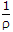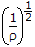# Online Electronics and Communication Engineering Test - Electronic Devices and Circuits TestLoading Test...

Instruction:

• This is a FREE online test. DO NOT pay money to anyone to attend this test.
• Total number of questions : 20.
• Time alloted : 30 minutes.
• Each question carry 1 mark, no negative marks.
• DO NOT refresh the page.
• All the best :-).

1.

In which of the following is the width of junction barrier very small?

A.
 Tunnel diodeB.
 Photo diodeC.
 PIN diodeD.
 Schottky diodeExplanation:

Schottky diode has very small depletion layer.

2.

An electron rises through a voltage of 100 V. The energy acquired by it will be

A.
 100 eVB.
 100 joulesC.
 (100)1.2 eVD.
 (100)1.2 joulesExplanation:

When an electron rises through 1 V, energy = 1 eV.

3.

Assertion (A): When Diode used as rectifier the reverse breakdown voltage should not be exceeded.

Reason (R): A high inverse voltage can destroy a p-n junction.

A.
 Both A and R are true and R is correct explanation of AB.
 Both A and R are true but R is not a correct explanation of AC.
 A is true but R is falseD.
 A is false but R is trueExplanation:

PIV is an important parameter in rectifier operation.

4.

At room temperature kT = 0.03 eV.

A.
 TrueB.
 False5.

An extrinsic semiconductor sample has 6 billion silicon atoms and 3 million pentavalent impurity atoms. The number of electrons and holes is

A.
 3 million eachB.
 6 billion eachC.
 3 million free electrons and very small number of holesD.
 3 million holes and very small number of free electronsExplanation:

When pentavalent impurity is added, the number of fresh electrons is very large as compared to number of holes.

6.

Consider the following statement: At finite temperature, magnetic dipoles in a material are randomly oriented giving low magnetization. When magnetic field H is applied, the magnetization?

1. Increases with H
2. Decreases with H
3. Decreases with temp for constant H
Which of the statement given above is/are correct?

A.
 1B.
 2C.
 2, 3D.
 1 and 37.

Which one of the following gain equations is correct for a MOSFET common-source amplifier?
(gm is mutual conductance, and RD is load resistance at the drain)

A.
 AV = gm/(1 - RD)B.
 AV = gm/RDC.
 AV = gm/(1 + RD)D.
 AV = RD/gm8.

The amount of time between the creation and disappearance of a hole in an intrinsic semiconductor material is called

A.
 life cycleB.
 recombination timeC.
 life timeD.
 half life9.

An intrinsic semiconductor (intrinsic electron density = 1016 m-3) is deped with donors to a level of 1022 m-3. What is the hole density assuming all donors to be ionized?

A.
 107 m-3B.
 108 m-3C.
 1010 m-3D.
 106 m-310.

Lowest noise can be expected in case of

A.
 carbon composition resistorsB.
 carbon film resistorsC.
 tin oxide resistorsD.
 metal film resistors11.

EG for silicon is 1.12 eV and that for germanium is 0.72 eV. Therefore it can be concluded that

A.
 more number of electron-hole pairs will be generated in silicon than in germanium at room temperatureB.
 less number of electron hole pairs will be generated in silicon than in germanium at room temperatureC.
 equal number of electron-hole pairs will be generated in both at lower temperaturesD.
 equal number of electron-hole pairs will be generated in both at higher temperatures12.

Fill in the suitable word in the blanks is the following questions. The electron in the outermost orbit is called __________ electron.

A.
 valenceB.
 covalentC.
 acceptorD.
 donor13.

Photoelectric emitters in photo tubes are generally made of

A.
 alkali metalsB.
 metalsC.
 semiconductorsD.
 metal and semiconductors14.

Before doping the semiconductor material is

A.
 dehydratedB.
 heatedC.
 hardenedD.
 purified15.

Due to the formation of Schottky defects the density of the crystal

A.
 increases slightlyB.
 increases appreciablyC.
 decreases slightlyD.
 decreases appreciably16.

Hall coefficient KH and charge density r are related as

A.
 KH =B.
 KH = rC.
 KH =D.
 KH = (r)1.217.

The equivalent circuit of an ideal diode is

A.
 a charging condenserB.
 a discharging condenserC.
 a switchD.
 a resistor18.

Piezoelectric quartz crystal resonators find application where

A.
 signal amplification is requiredB.
 rectification of the signal is requiredC.
 signal frequency control is requiredD.
 modulation of signal is required19.

The number of protons in a silicon atom is

A.
 32B.
 28C.
 14D.
 420.

Each cell of a static Random Access memory contains

A.
 6 MOS transistorB.
 4 MOS transistor, 2 capacitorC.
 2 MOS transistor, 4 capacitorD.
 1 MOS transistor and 1 capacitor